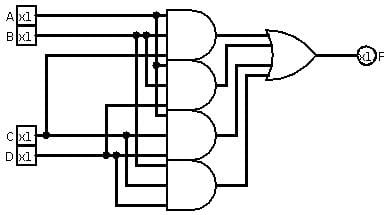# Majority function

Updated on
Covid-19In Boolean logic, the majority function (also called the median operator) is a function from n inputs to one output. The value of the operation is false when n/2 or more arguments are false, and true otherwise. Alternatively, representing true values as 1 and false values as 0, we may use the formula

## Contents

Majority ( p 1 , , p n ) = 1 2 + ( i = 1 n p i ) 1 / 2 n .

The "−1/2" in the formula serves to break ties in favor of zeros when n is even. If the term "−1/2" is omitted, the formula can be used for a function that breaks ties in favor of ones.

## Boolean circuits

A majority gate is a logical gate used in circuit complexity and other applications of Boolean circuits. A majority gate returns true if and only if more than 50% of its inputs are true.

For instance, in a full adder, the carry output is found by applying a majority function to the three inputs, although frequently this part of the adder is broken down into several simpler logical gates.

Many systems have triple modular redundancy; they use the majority function for majority logic decoding to implement error correction.

A major result in circuit complexity asserts that the majority function cannot be computed by AC0 circuits of subexponential size.

## Monotone formulae for majority

For n = 1 the median operator is just the unary identity operation x. For n = 3 the ternary median operator can be expressed using conjunction and disjunction as xy + yz + zx. Remarkably this expression denotes the same operation independently of whether the symbol + is interpreted as inclusive or or exclusive or.

For an arbitrary n there exists a monotone formula for majority of size O(n5.3). This is proved using probabilistic method. Thus, this formula is non-constructive. However, one can obtain an explicit formula for majority of polynomial size using a sorting network of Ajtai, Komlós, and Szemerédi.

The majority function produces "1" when more than half of the inputs are 1; it produces "0" when more than half the inputs are 0. Most applications deliberately force an odd number of inputs so they don't have to deal with the question of what happens when exactly half the inputs are 0 and exactly half the inputs are 1. The few systems that calculate the majority function on an even number of inputs are often biased towards "0"—they produce "0" when exactly half the inputs are 0 -- for example, a 4-input majority gate has a 0 output only when two or more 0's appear at its inputs. In a few systems, a 4-input majority network randomly chooses "1" or "0" when exactly two 0's appear at its inputs.

## Properties

For any x, y, and z, the ternary median operator 〈x, y, z〉 satisfies the following equations.

• x, y, y〉 = y
• x, y, z〉 = 〈z, x, y
• x, y, z〉 = 〈x, z, y
• 〈〈x, w, y〉, w, z〉 = 〈x, w, 〈y, w, z〉〉
• An abstract system satisfying these as axioms is a median algebra.

## References

Similar Topics
Thicker than Water (2005 film)
Damon Andrews
Ryan Shane
Topics